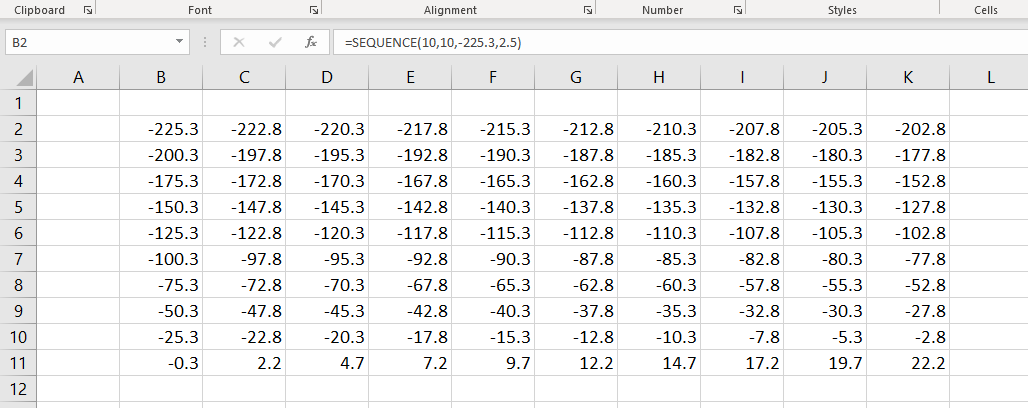# SEQUENCE FunctionThe Excel SEQUENCE function is a DYNAMIC ARRAY FUNCTION that can generate a list or array of sequential numbers. The array can be one dimensional or two-dimensional.

### Function Type

Math and trigonometry

### Purpose

Generate an array of sequential numbers

### Return value

An array of sequential numbers

### Syntax

` =SEQUENCE (rows, [columns], [start], [step])`

### Arguments

rows – number of rows of the array

columns – [optional] Number of columns of array

start – [optional] starting value. Default value is 1

step – [optional] Increment between each value. Default value is 1

### Examples

#### Example 1

Use the formula to create a sequence of numbers from 1 to 10

`=SEQUENCE(10)`

#### Example 2

To create an array of size 10 x 10 containing a sequence of numbers from 1 to 100 use the following formula

`=SEQUENCE(10,10)`

#### Example 3

Formula to create an array of size 5 x 7 containing a sequence of numbers starting from 253

`=SEQUENCE(5,7,253)`

#### Example 4

Formula to create an array of size 8 x 8 containing a sequence of numbers having a difference of 3, starting from 1500.

`=SEQUENCE(8,8,1500,3)`

#### Example 5

The SEQUENCE function can also handle negative numbers and decimals.

Formula to create an array of size 10 x 10 containing a sequence of numbers having a difference of 2.5, starting from -7.8.

`=SEQUENCE(10,10,-225.3,2.5)`